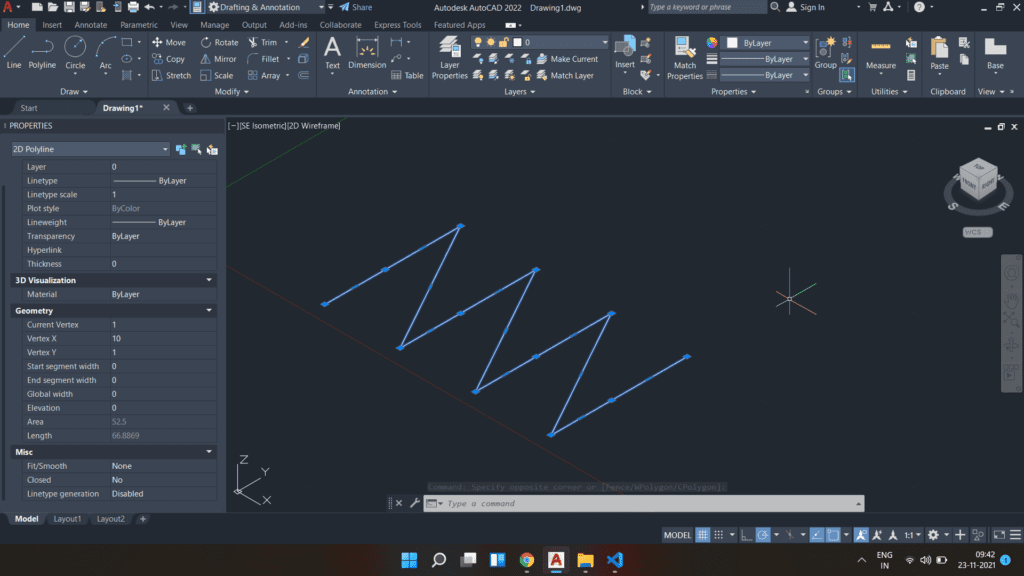In this blog post, we are going to look at how we can work with 3D mesh objects in AutoCAD using the pyautocad module. If we want, we can use the pywin32 module too to work with 3D mesh.

## Significance of 3D mesh representation

The 3D mesh can be used in several industries for different purposes.

Being a civil engineer, I can definitely say, while working as a land surveyor the 3D mesh can be very helpful while representing contour points. It can also be used in construction defects by doing an inspection of coordinates of every small element of a structure.

It can be used in the mechanical industry while working with machine design.

Nowadays, we also use the mesh model for facial recognition. Here it can furthermore e.g. be used to detect changes in facial expressions.

There are more such use cases of 3D mesh that play a very important role in many industries.

## Initiating the script in Python using pyautocad or pywin32

We can set up our work environment by using the pyautocad module as given below:

``````from pyautocad import Autocad, aDouble

Alternatively, we can also use win32com.client to get the things running as per the following script:

``````from win32com.client import *
import pythoncom

return win32com.client.VARIANT(pythoncom.VT_ARRAY | pythoncom.VT_R8, (argv))``````

To draw a 3D mesh onto the AutoCAD template it takes a very small command with a few parameters.

The syntax of the command is as stated below:

Here, M & N takes an integer input ranging from 2-256 representing the size of the array in (or the number of vertices along) both M & N directions.

The PointsMatrix represents the array of doubles. It is the same matrix that we e.g. on when creating polylines with pyautocad.

Let’s create one such mesh model using the existing example as provided in the Autodesk documentation. I will take the PointsMatrix as provided in the documentation.

``````pmatrx = aDouble(10, 1, 3, 10, 5, 5, 10, 10, 3, 15, 1, 0, 15, 5, 0, 15, 10, 0, 20, 1, 0, 20, 5, -1, 20, 10, 0, 25, 1, 0, 25, 5,  0, 25, 10, 0)

In this code, we have provided 12 coordinate points in the PointsMatrix.

Also, we want 4 vertices along the M direction and 3 vertices along the N direction.

## Analysis of the newly created 3D mesh

As we can see from Figures 2.1 & 2.2, we have 4 vertices in the M direction & 3 vertices along N as passed in the command.

Before drawing the same we have visualized that the vertices shall be in this format. i.e.

Else, if we try to draw a mesh with a polyline using the same set of coordinate points, it won’t result in a mesh rather simply connecting the points in given series forming a zigzag pattern.

``pl = acad.model.AddPolyline(pmatrx)``Figure 3.2: Polyline using the same set of coordinates as used for drawing the 3D mesh

We can also see how the mesh model looks if we use the realistic view rather than the 2D wireframe. This will clearly show us the depressions or humps if any in the given mesh model.

As mentioned above, from Figure 3.3, the depression can be clearly seen in the right half of the mesh also the central portion of the left half is protruding out at the boundary.

## Properties of a 3D mesh using pyautocad

We have some mesh-specific methods that can be used to fetch the properties of a given mesh other than a few common methods that we use in AutoCAD operations.

``````print("Coordinates of the mesh:", end='')
print(mesh1.Coordinates)
print("Is mesh one is closed in M direction: " + str(mesh1.MClose))
print("Density of mesh in M direction: " + str(mesh1.MDensity))
print("Number of vertices in M direction: " + str(mesh1.MVertexCount))
print("Is mesh one is closed in N direction: " + str(mesh1.NClose))
print("Density of mesh in N direction: " + str(mesh1.NDensity))
print("Number of vertices in N direction: " + str(mesh1.NVertexCount))

O/p:

Coordinates of the mesh:(10.0, 1.0, 3.0, 10.0, 5.0, 5.0, 10.0, 10.0, 3.0, 15.0, 1.0, 0.0, 15.0, 5.0, 0.0, 15.0, 10.0, 0.0, 20.0, 1.0, 0.0, 20.0, 5.0, -1.0, 20.0, 10.0, 0.0, 25.0, 1.0, 0.0, 25.0, 5.0, 0.0, 25.0, 10.0, 0.0)
Is mesh one is closed in M direction: False
Density of mesh in M direction: 0
Number of vertices in M direction: 4
Is mesh one is closed in N direction: False
Density of mesh in N direction: 0
Number of vertices in N direction: 3``````